# A Matlab package for georectifying oblique digital images (g rect)

(diff) ← Older revision | Latest revision (diff) | Newer revision → (diff)

## Introduction

This toolbox contains a series of Matlab functions for georectifying highly oblique digital images. This is useful in oceanography for obtaining quantitative information on sea-surface patterns such as those induced by internal waves, fronts or sea-ice.

Although this toobox was initially developed to georectify geophysical images it can be used just as well for rectifying laboratory images. The following images show examples of such image rectifications.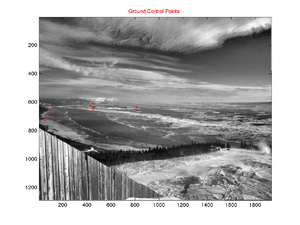Example of a geophysical photo showing sea-ice distribution in the St. Lawrence Estuary.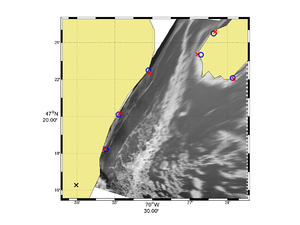Same as previous photo once georectified.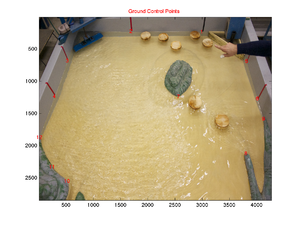Example of a laboratory basin photo.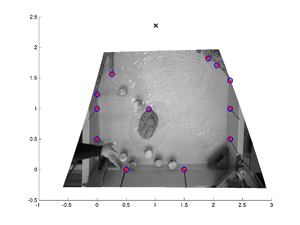Same as previous once georectified.

## How to get the package

Once expanded the g_rect package is organized as follow:

• g_rect_package
• src (the source codes)
• g_rect (contains the main georectification functions)
• g_calib (contains camera calibration functions)
• g_stabilize (contains image stabilization functions)
• Examples
• Field (a field example)
• Lab (a lab example)

The main georectification functions are found within the g_rect directory. The other two directories are for more advanced processing if the camera needs to be calibrated (g_calib) or if you need to stabilize (i.e. removing small movements between successive images) a series of images (g_stabilize). You may not need to play at all with these.

## g_rect: Quick Start

For a quick start let's only play with the main function called g_rect.m found under the g_rect directory. First you should set this directory into your Matlab path (File -> Set Path).

Then go with Matlab into the directory Examples/Field. You will find there an image (IMG_6614.JPG) and a file named parameters.dat. This is the main input parameter file that you need to edit for a given image or for a given series of images.

This input parameter file looks like this:

### Input file: parameters.dat

```% I/O information
imgFname      =  'IMG_6614.JPG';   % This is the reference image
firstImgFname =  'IMG_6614.JPG';  % This is just to indicate the first image of a sequence
lastImgFname  =  'IMG_6614.JPG'; % This is just to indicate the last image of a sequence
outputFname   =  'g_rect.mat';      % This is the output filename where the new coordinates will be stored.

% Field or lab case situation.
% Set field = true for field situation and field = false for lab situation.
% Lab situation does not expect to work with latitude and longitude but simply with meters.
field = true;

% Camera position
% lat/lon for field situation
% meter for lab situation
LON0 = -70.6010167;
LAT0 =  47.2713000;

% Offset from center of the principal point (generally zero)
ic = 0;
jc = 0;

% Parameters
hfov =      45.0;     % Field of view of the camera
lambda =    2;        % Dip angle above vertical (e.g. straight down = 90, horizontal = 0)
phi =       0.0;      % Tilt angle (generally close to 0)
H =         720;      % Camera altitude
theta =     70.0;     % View angle clockwise from North (e.g. straight East = 90)

% Uncertainty in parameters. Set the uncertainty to 0.0 for fixed parameters.
dhfov =     20.0;
dlambda =   10.0;
dphi =      5.0;
dH =        0.0;
dtheta =    20.0;

% Order of the polynomial correction (0, 1 or 2)
polyOrder = 1;

% To save memory calculation can be done in single precision.
% For higher precision set the variable 'precision' to 'double'.
precision = 'double';

% Ground Control Points (GCP).
% The data must come right after the gcpData = true
gcpData = true;
360  829  -70.561367  47.303783
54  719  -70.54500   47.335
99  661  -70.505     47.375
452  641  -70.435     47.389
429  633  -70.418     47.408
816  644  -70.393     47.368
```

This file contains all parameters required to perform a georectification. You can add spaces and comments as you wish within this file. One specify format needs however to be respected. The ground control points (gcp) must appear right after the variable gcpData = true; These ground control points represent the lon-lat position associated with some image coordinates.

If you know perfectly some of the camera parameters (e.g. the field of view) you simply have to set the uncertainty of this parameter to zero (e.g. dfov = 0). Otherwise, for other parameters with some uncertainty the minimization algorithm will search within the given uncertainty to find the best parameters values that gives a best fit between the image control points once georectified and the ground control points (see Bourgault, 2008, for details).

To run the georectification, all you have to do in principle is to edit this file and to type in Matlab g_rect at the command line. You will then be prompted for the name of the input parameter file (it does not have to be called parameters.in). You image will appear with the GCPs as well as a table re-listing all of your parameters. If you're ok with this type enter.

You will then see the algorithm searching the parameter space and will eventually return the result for the set of parameters found. A file will be created, named accoring to the name given in the variable outputFname. This is the main result file that contain all important variables. The most important matrices created are named LAT and LON. These two matrices have the same size as your original image and provides the latitude and longitude of every associated pixel. You can then make a figure using for example the command pcolor (or m_pcolor if you use the m_map package) with LON and LAT as the arguments for the position and a third argument for the pixel intensity which could simply be the mean of the RGB channel if you want to reduce a color image to a grayscale image. Fancier color representation could also be done but this is up to you.

The g_rect package works best with the m_map package installed.

## g_calib

Camera calibration could be done if required. All files needed are found in the g_calib folder. The package comes from this site that you should consult for information on how to perform a camera calibration: http://www.vision.caltech.edu/bouguetj/calib_doc/

Essentially, a camera calibration removes distortion associated with a lens as shown by comparing the images below.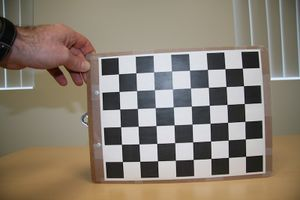Example of an original image showing obvious distortion. The checkboard should be flat but curvature is seen.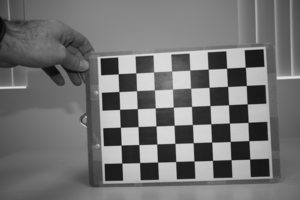The same image once calibrated.

Note that this step is not always required for two main reasons. Firstly, it could be that the camera and lens you use has little acceptable distortion. Secondly, the algorithm in g_rect provides a polynomial correction (of order 1 or 2) to the image if you have enough ground control points (it is the variable name polyOrder in the input parameter file). This is an indirect way of correcting for lens distortion that is often acceptable.

## g_stablilize

Generally, sequences of images are collected from a fixed tripod. For example, you may want to observe the movement of sea-ice over a semi-diurnal cycle at a sample rate of 1 image per minute (roughly 750 images). If the tripod does not move during the collection then the georectification procedure described above with g_rect only needs to be applied to one image. The coordinate matrices LAT and LON can then be applied to all images of the sequence.

In practice, the camera moves for many reasons. It may be because you need to replace the batteries, because you touched the camera by mistake, because you need to change a camera setting half-way through the experiment or, if you're outside, because wind gusts induce small but perceptible camera movements. If you don't correct for these movements, the sequence will be jumpy if you make a movie with it. This may lead to inaccurate measurements if you need to compare two frames, say for calculating ice drift velocities.

To fix this problem you could apply a new georectification to every images that has moved. This is however not practical if, for example, camera movements were randomly induced by wind gusts. In some windy situations, just about every image show small but perceptible camera movements. You don't want to apply the georectification 750 times.

This is when camera stabilization becomes very useful. The idea is to choose one reference image, generally the first image of the sequence, and to geometrically transform all other images, for translation, scale and rotation, to match the reference image as close as possible. This step is done before doing the georectification. However, for this to work we need to tell the algorithm which parts of the image are not supposed to have moved. The algorithm cannot tell by itself whether it is the camera that moved of the ice we are observing. These portions of image are called region of interest (ROI). Details of this procedure are described by Farid and Woodward (2007). The Matlab algorithm found in Farid and Woodward (2007) have been adapted here.

The folder g_stabilize contains a series of Matlab functions for performing the image stabilization. However, you need to be familiar with only the following two functions:

### g_roi.m

This function is a utility that helps you determine region of interest. To use it you simply have to call the function with your reference image filename such as

```g_roi('IMG_1.jpg")
```

and follow the instructions. Essentially, you will be asked to make polygons around regions of interests (roi). These are regions in your image that don't move. Try not to take moving patterns on the water or the sky that has moving clouds.

This function only puts 1s in ROIs and 0s everywhere else. Once you're done a file names roi.mat is created. The blue and red image below shows the ROI image corresponding to the image #1. This file is then needed by the next function that performs the actual stabilization.

### g_stabilize.m

Once you have created the roi.mat file you simply type, in principle, the following command:

```g_stabilize(root_img_name,ext,id_img_ref,id_img_first,id_img_last)
```

where

```root_img_name is the root of the images name (e.g. IMG_;
ext is the images extension (e.g. 'png' or 'jpg';
id_img_ref is the number that identifies the reference image;
id_img_first is the number of the first image of the sequence you want to stabilize;
id_img_last is the number of the last image of the sequence you want to stabilize;
```

This will recreate a series of images with the same name but with the suffix _stable added to your image filename. The original images are conserved.

### Remark

Because of rapidly changing light conditions outside, the stabilize algorithm may not work as you would hope. This is because region of interests are often subject to moving cloud shadows that may fool the algorithm. In some cases it may be necessary to filter first you image and to apply equalizers in order to minimize as much as possible such motions. In general, the algorithm does quite a good job. You can appreciate the effect by downloading and comparing IMG_1.JPG with IMG_58.JPG and IMG_58_stable.JPG below.

 File:IMG 1.JPG Example of the first image of a sequence (image #1). All subsequent images can be stabilized using this image as the reference. File:IMG 58.JPG Image #58 shows important differences in the view point compared to the first image. It was very windy that day and the tripod moved with wind gusts.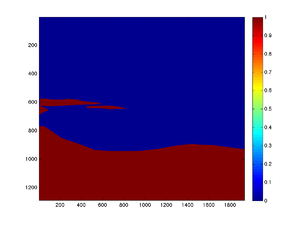Region of interest (ROI) needed for the stabilization algorithm. This can be done with the function g_roi.m File:IMG 58 stable.JPG Image #58 once stabilized relative to image #1.